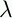September 22, 1999 Perl and the Lambda Calculus Slide #27

# Arithmetic

• Let's make an addition function now

• It will look something like this:

```        ADD =mn.(IF (IS_ZERO m) n (ADD (PRED m) (SUCC n)))
```
• Whoops!

• How can we use ADD in its own definition?

 NextCopyright © 1999 M-J. Dominus